##### Introduction

Here I will describe how to show data in nested datagrid using C# winforms

This is applicable to those cases where we have to display master and child content / rows.

Example Student Table

Here in this example we have list of Students  and inside we have details of student marks

##### Student Roll number

1

Devesh omar

0302713014

 SubjectID SubjectName Marks 123 Physics 75 321 chemistry 99 555 Maths 100

2

Nikhil

03027130145

 SubjectID SubjectName Marks 123 Physics 72 321 C# 100 555 Maths 100

Our purpose is to display this type of data using C#  Winforms

Steps and Code:

1. First we need to create Master and child tables.
2. To identify the relationship between tables we should have Primary and Foreign Keys in tables
3. Here we are creating datatable for master and child tables.
4. Master table  (student)
`                //Parent table            DataTable dtstudent = new DataTable();            dtstudent.Columns.Add("Student_ID", typeof(int));            dtstudent.Columns.Add("Student_Name", typeof(string));            dtstudent.Columns.Add("Student_RollNo", typeof(string));`

1. Child Table (Student Marks)
`//Child table            DataTable dtstudentMarks = new DataTable();            dtstudentMarks.Columns.Add("Student_ID", typeof(int));            dtstudentMarks.Columns.Add("Subject_ID", typeof(int));            dtstudentMarks.Columns.Add("Subject_Name", typeof(string));            dtstudentMarks.Columns.Add("Marks", typeof(int)); `

f.  Understanding Relation ship between Master and child table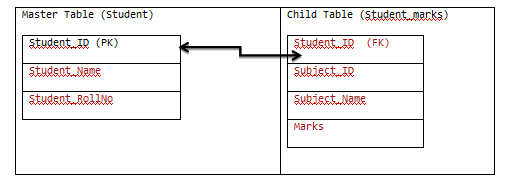g.  In brief

dtstudent.StudentID = dtstudentMarks.StudentID

Adding Rows to Master table (Student Table)

`//Adding Rows             dtstudent.Rows.Add(111, "Devesh", "03021013014");            dtstudent.Rows.Add(222, "ROLI", "0302101444");            dtstudent.Rows.Add(333, "ROLI Ji", "030212222");            dtstudent.Rows.Add(444, "NIKHIL", "KANPUR"); `

i.  Adding Row to Child table (Student Marks table)

`   // data for devesh ID=111            dtstudentMarks.Rows.Add(111, "01","Physics", 99);            dtstudentMarks.Rows.Add(111, "02","Maths", 77);            dtstudentMarks.Rows.Add(111, "03","C#", 100);            dtstudentMarks.Rows.Add(111, "01","Physics", 99);              //data for ROLI ID=222            dtstudentMarks.Rows.Add(222, "01", "Physics", 80);            dtstudentMarks.Rows.Add(222, "02", "English", 95);            dtstudentMarks.Rows.Add(222, "03", "Commerce", 95);            dtstudentMarks.Rows.Add(222, "01", "BankPO", 99);`

Adding master and child table to dataset.

`DataSet dsDataset = new DataSet();  dsDataset.Tables.Add(dtstudent);  dsDataset.Tables.Add(dtstudentMarks);`

Defining relationship between Master and child table

`DataRelation Datatablerelation = new DataRelation("DetailsMarks", dsDataset.Tables.Columns, dsDataset.Tables.Columns,true);            dsDataset.Relations.Add(Datatablerelation);`

l.  Adding Datagrid control in winforms

As per screen below we can add datagrid to winforms

Right click in tool bar -> choose itemm.    Binding data

`dataGrid1.DataSource = dsDataset.Tables; `

n.      All code together

`private void Form1_Load(object sender, EventArgs e)        {            //Parent table            DataTable dtstudent = new DataTable();            // add column to datatable              dtstudent.Columns.Add("Student_ID", typeof(int));            dtstudent.Columns.Add("Student_Name", typeof(string));            dtstudent.Columns.Add("Student_RollNo", typeof(string));                        //Child table            DataTable dtstudentMarks = new DataTable();            dtstudentMarks.Columns.Add("Student_ID", typeof(int));            dtstudentMarks.Columns.Add("Subject_ID", typeof(int));            dtstudentMarks.Columns.Add("Subject_Name", typeof(string));            dtstudentMarks.Columns.Add("Marks", typeof(int));                         //Adding Rows             dtstudent.Rows.Add(111, "Devesh", "03021013014");            dtstudent.Rows.Add(222, "ROLI", "0302101444");            dtstudent.Rows.Add(333, "ROLI Ji", "030212222");            dtstudent.Rows.Add(444, "NIKHIL", "KANPUR");                 // data for devesh ID=111            dtstudentMarks.Rows.Add(111, "01","Physics", 99);            dtstudentMarks.Rows.Add(111, "02","Maths", 77);            dtstudentMarks.Rows.Add(111, "03","C#", 100);            dtstudentMarks.Rows.Add(111, "01","Physics", 99);              //data for ROLI ID=222            dtstudentMarks.Rows.Add(222, "01", "Physics", 80);            dtstudentMarks.Rows.Add(222, "02", "English", 95);            dtstudentMarks.Rows.Add(222, "03", "Commerce", 95);            dtstudentMarks.Rows.Add(222, "01", "BankPO", 99);               DataSet dsDataset = new DataSet();            //Add two DataTables  in Dataset             dsDataset.Tables.Add(dtstudent);            dsDataset.Tables.Add(dtstudentMarks);                        DataRelation Datatablerelation = new DataRelation("DetailsMarks", dsDataset.Tables.Columns, dsDataset.Tables.Columns, true);            dsDataset.Relations.Add(Datatablerelation);            dataGrid1.DataSource = dsDataset.Tables;         }`

o. Running code

We will get following screen having expandable rows in datagrid.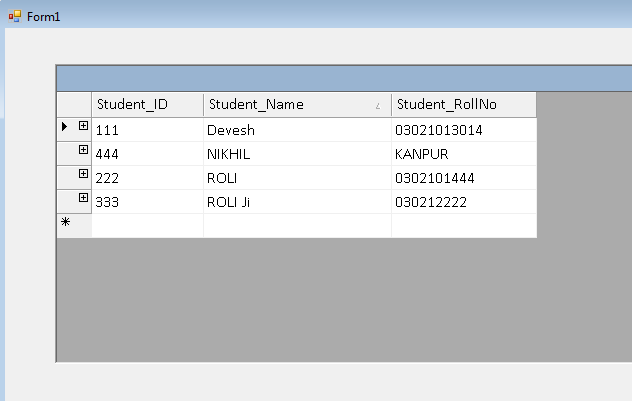p.      Expanding row to see details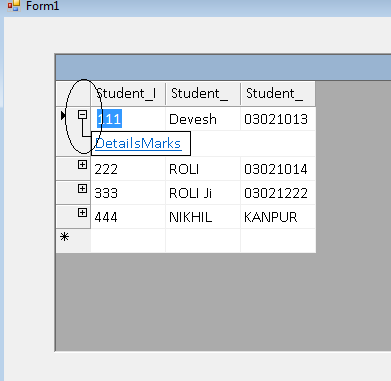q.      Click on DetailsMarks to get Marks details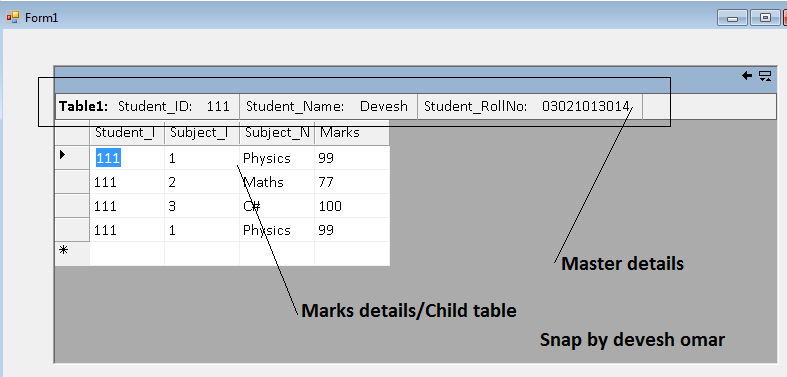r.        Details screen for student_Id=222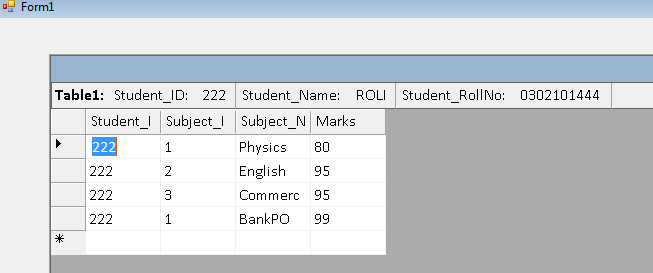s.       Go to back for Master details

Click on highlighted back button to go master detail form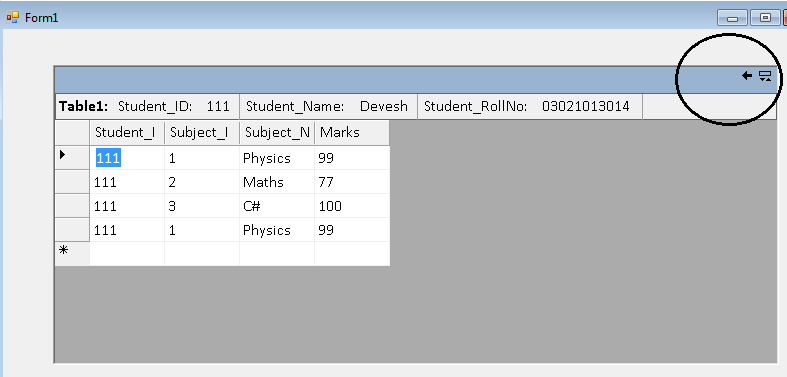t.        Main form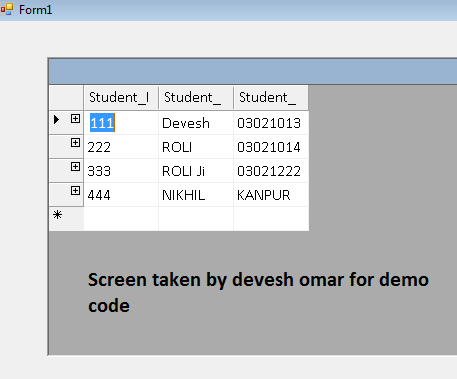Conclusion

We have learned how to show data in datagrid with master and child relationship.

Modified On Dec-10-2019 11:05:28 PM
1.Keep Writing Devesh.... Good
1.hi dear friends
fortunately i could use this article for my program but i had some problems .because i have been using data grid view in my program that every cell of that filled  by a value after computations when my program had  been running .so i did not use data source for showing data in data grid view .in addition, at the moment , i want to connect my data grid view with a datatable.